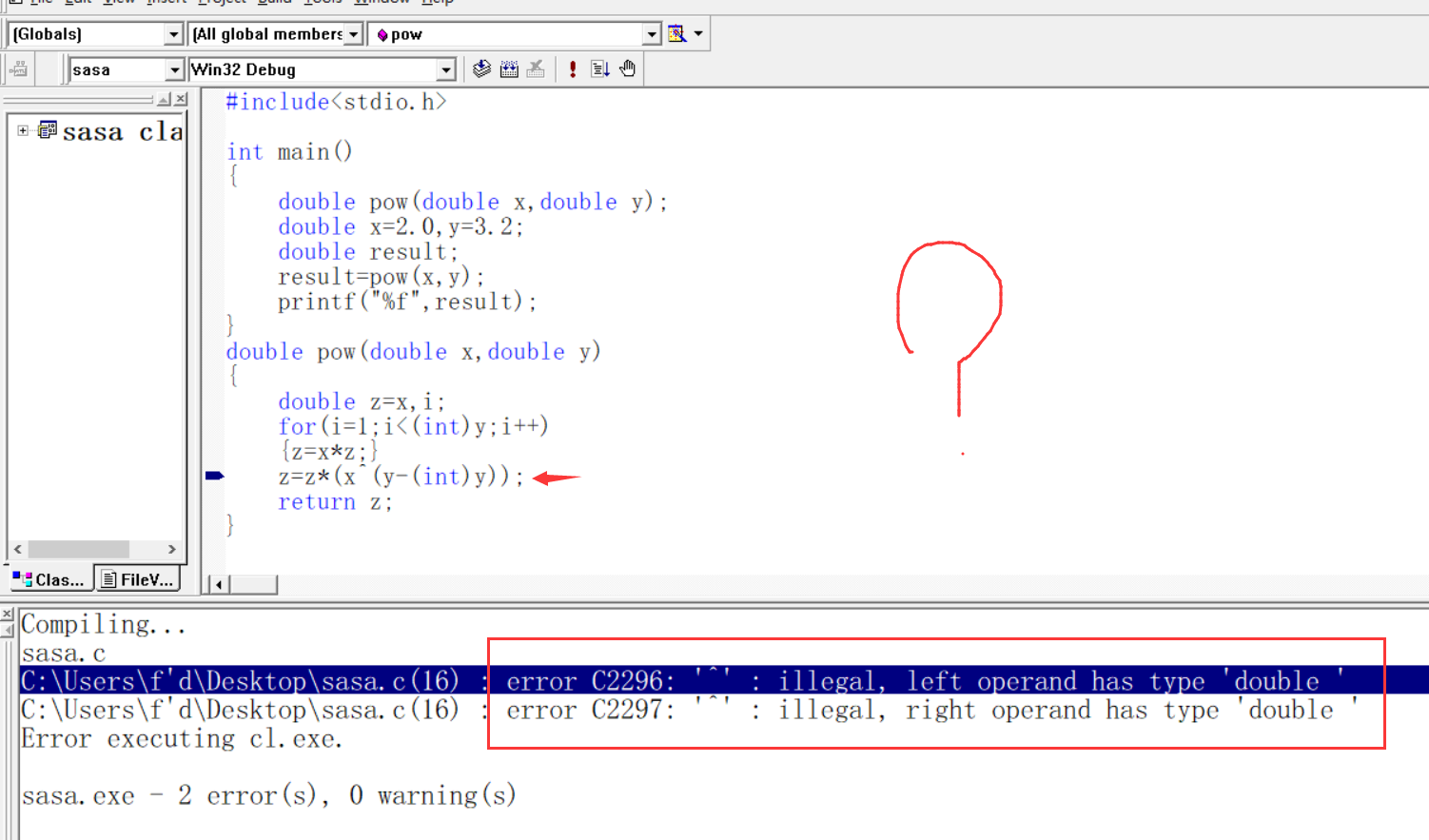2021-08-27 12:01

# C语言自编程序实现pow（）函数功能时程序遇到一个问题！求解``````
#include<stdio.h>

int main()
{
double pow(double x,double y);
double x=2.0,y=3.2;
double result;
result=pow(x,y);
printf("%f",result);
}
double pow(double x,double y)
{
double z=x,i;
for(i=1;i<(int)y;i++)
{z=x*z;}
z=z*(x^(y-(int)y));
return z;
}

``````
• 收藏